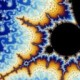# Superb Mandelbrot Set On WikipediaSame building different angle on Fuxing North Rd.(復興北路)

These are superb images I found of a Mandelbrot Set on wikipedia. They were so gorgeous and reminded me of the time when I was studying Complex Mathematical Analysis that I just had to post them.

[ A small digression:

At the time, I was obsessed with Chaos Theory and complex mathematics.

One of the things that really got me out of mathematics was that it was all too abstract; all the concepts were not grounded in palpable reality.

And I wanted to be on Earth…

Digression ends here.]

A Mandelbrot Set is a fractal.

The Mandelbrot set is a fractal that has become popular outside of mathematics both for its aesthetic appeal and a complicated structure arising from a simple definition. Benoît Mandelbrot and others worked hard to communicate this area of mathematics to the public.

A fractal is:

In colloquial usage, a fractal is “a rough or fragmented geometric shape that can be subdivided in parts, each of which is (at least approximately) a reduced-size copy of the whole”. The term was coined by Benoît Mandelbrot in 1975 and was derived from the Latin fractus meaning “broken” or “fractured”.

A fractal as a geometric object generally has the following features:

• It has a fine structure at arbitrarily small scales.
• It is too irregular to be easily described in traditional Euclidean geometric language.
• It is self-similar (at least approximately or stochastically).
• It has a Hausdorff dimension that is greater than its topological dimension (although this requirement is not met by space-filling curves such as the Hilbert curve).
• It has a simple and recursive definition.

Because they appear similar at all levels of magnification, fractals are often considered to be infinitely complex (in informal terms). Natural objects that approximate fractals to a degree include clouds, mountain ranges, and lightning bolts. However, not all self-similar objects are fractals—for example, the real line (a straight Euclidean line) is formally self-similar but fails to have other fractal characteristics.The images were produced by Wolfgang Beyer with the Ultra Fractal 3 program:

mandelZoom00MandelbrotSet {
fractal:
title=”mandel zoom 00 mandelbrot set” width=2560 height=1920 layers=1
credits=”WolfgangBeyer;8/21/2005″
layer:
method=multipass caption=”Background” opacity=100
mapping:
center=-0.7/0 magn=1.3
formula:
maxiter=50000 filename=”Standard.ufm” entry=”Mandelbrot” p_start=0/0
p_power=2/0 p_bailout=10000
inside:
transfer=none
outside:
density=0.42 transfer=log filename=”Standard.ucl” entry=”Smooth”
p_power=2/0 p_bailout=128.0
smooth=yes rotation=29 index=28 color=6555392 index=92 color=13331232
index=196 color=16777197 index=285 color=43775 index=371 color=3146289
opacity:
smooth=no index=0 opacity=255
}## Author: range

I'm mathematician/IT strategist/blogger from Canada living in Taipei.

Posted on Categories photos, technology